Next: 14.5 Inverse Function Theorems Up: 14. The Inverse Function Previous: 14.3 Inverse Functions   Index

# 14.4 The Exponential Function

14.29   Example. We will now derive some properties of the inverse function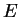of the logarithm.

We have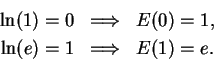For all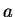and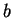in R,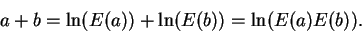If we applyto both sides of this equality we get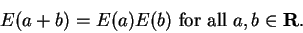For all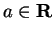we have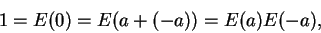from which it follows that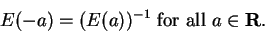Ifand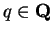we have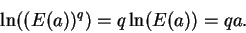If we applyto both sides of this identity we get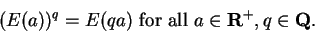In particular,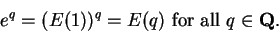(14.30)

Now we have defined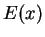for all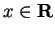, but we have only defined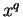when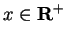and. (We know what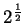is, but we have not defined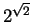.) Because of relation (14.30) we often write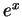in place of.is called the exponential function, and is written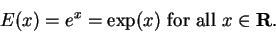We can summarize the results of this example in the following theorem:

14.31   Theorem (Properties of the exponential function.) The exponential function is a function from R onto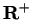. We have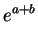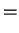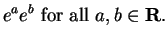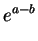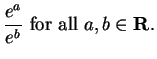(14.32)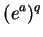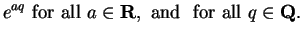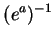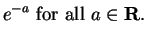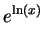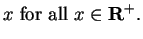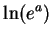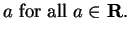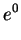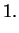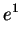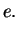(14.33)

Proof: We have proved all of these properties except for relation (14.32). The proof of (14.32) is the next exercise.

14.34   Exercise. Show that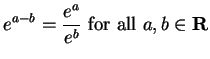.

14.35   Exercise. Show that if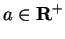and, then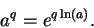14.36   Definition (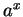.) The result of the last exercise motivates us to make the definition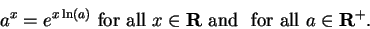14.37   Exercise. Prove the following results: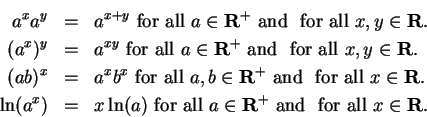Next: 14.5 Inverse Function Theorems Up: 14. The Inverse Function Previous: 14.3 Inverse Functions   Index
Ray Mayer 2007-09-07